Share

RD Sharma solutions for Class 9 Mathematics chapter 14 - Areas of Parallelograms and Triangles

Mathematics for Class 9 by R D Sharma (2018-19 Session)

RD Sharma Mathematics Class 9 by R D Sharma (2018-19 Session)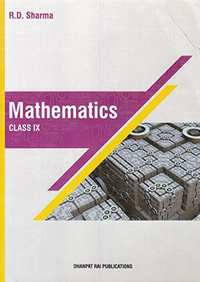Chapter 14: Areas of Parallelograms and Triangles

Ex. 14.10Ex. 14.20Ex. 14.30Others

Chapter 14: Areas of Parallelograms and Triangles Exercise 14.10 solutions [Page 3]

Ex. 14.10 | Q 1 | Page 3

Which of the following figures lie on the same base and between the same parallels. In such a case, write the common base and the two parallels.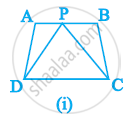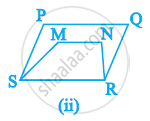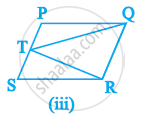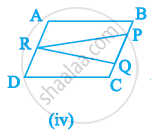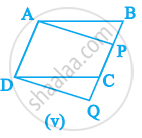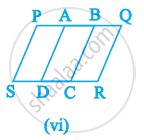Chapter 14: Areas of Parallelograms and Triangles Exercise 14.20 solutions [Page 15]

Ex. 14.20 | Q 1 | Page 15

In fig below, ABCD is a parallelogram, AE ⊥ DC and CF ⊥ AD. If AB = 16 cm, AE = 8
cm and CF = 10 cm, find AD.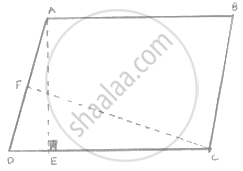Ex. 14.20 | Q 2 | Page 15

In Q. No 1, if AD = 6 cm, CF = 10 cm, and AE = 8cm, find AB.

Ex. 14.20 | Q 3 | Page 15

Let ABCD be a parallelogram of area 124 cm2. If E and F are the mid-points of sides AB and
CD respectively, then find the area of parallelogram AEFD.

Ex. 14.20 | Q 4 | Page 15

If ABCD is a parallelogram, then prove that
𝑎𝑟 (Δ𝐴𝐵𝐷) = 𝑎𝑟 (Δ𝐵𝐶𝐷) = 𝑎𝑟 (Δ𝐴𝐵𝐶) = 𝑎𝑟 (Δ𝐴𝐶𝐷) = 1/2 𝑎𝑟 (||𝑔𝑚 𝐴𝐵𝐶𝐷) .

Chapter 14: Areas of Parallelograms and Triangles Exercise 14.30 solutions [Pages 44 - 48]

Ex. 14.30 | Q 1 | Page 44

In the below figure, compute the area of quadrilateral ABCD.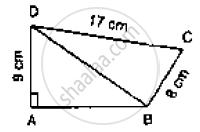Ex. 14.30 | Q 2 | Page 44

In the below figure, PQRS is a square and T and U are respectively, the mid-points of PS
and QR. Find the area of ΔOTS if PQ = 8 cm.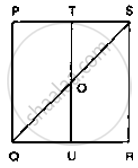Ex. 14.30 | Q 3 | Page 44

Compute the area of trapezium PQRS is Fig. below.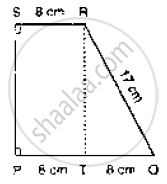Ex. 14.30 | Q 4 | Page 44

In the below fig. ∠AOB = 90°, AC = BC, OA = 12 cm and OC = 6.5 cm. Find the area of
ΔAOB.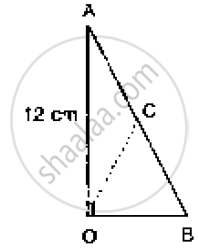Ex. 14.30 | Q 5 | Page 44

In the below fig. ABCD is a trapezium in which AB = 7 cm, AD = BC = 5 cm, DC = x cm,
and distance between AB and DC is 4cm. Find the value of x and area of trapezium ABCD.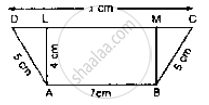Ex. 14.30 | Q 6 | Page 45

In the below fig. OCDE is a rectangle inscribed in a quadrant of a circle of radius 10 cm. If
OE = 2√5, find the area of the rectangle.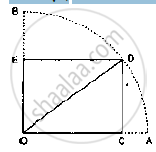Ex. 14.30 | Q 7 | Page 45

In the below fig. ABCD is a trapezium in which AB || DC. Prove that ar (ΔAOD) =
ar(ΔBOC).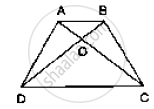Ex. 14.30 | Q 8 | Page 45

In the given below fig. ABCD, ABFE and CDEF are parallelograms. Prove that ar (ΔADE)
= ar (ΔBCF)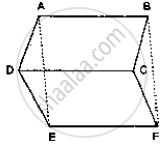Ex. 14.30 | Q 9 | Page 45

In the below Fig, ABC and ABD are two triangles on the base AB. If line segment CD is
bisected by AB at O, show that ar (Δ ABC) = ar (Δ ABD)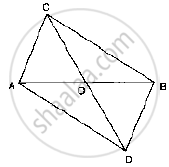Ex. 14.30 | Q 10 | Page 45

If AD is a median of a triangle ABC, then prove that triangles ADB and ADC are equal in
area. If G is the mid-point of median AD, prove that ar (Δ BGC) = 2 ar (Δ AGC).

Ex. 14.30 | Q 11 | Page 45

A point D is taken on the side BC of a ΔABC such that BD = 2DC. Prove that ar(Δ ABD) =

Ex. 14.30 | Q 12 | Page 46

ABCD is a parallelogram whose diagonals intersect at O. If P is any point on BO, prove
that:  (1) ar (ΔADO) = ar (ΔCDO)     (2) ar (ΔABP) = ar (ΔCBP)

Ex. 14.30 | Q 13 | Page 46

ABCD is a parallelogram in which BC is produced to E such that CE = BC. AE intersects
CD at F.
(i) Prove that ar (ΔADF) = ar (ΔECF)
(ii) If the area of ΔDFB = 3 cm2, find the area of ||gm ABCD.

Ex. 14.30 | Q 14 | Page 46

ABCD is a parallelogram whose diagonals AC and BD intersect at O. A line through O
intersects AB at P and DC at Q. Prove that ar (Δ POA) = ar (Δ QOC).

Ex. 14.30 | Q 15 | Page 46

In the below fig. D and E are two points on BC such that BD = DE = EC. Show that ar
(ΔABD) = ar (ΔADE) = ar (ΔAEC).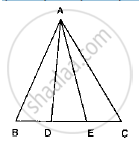Ex. 14.30 | Q 16 | Page 46

Diagonals AC and BD of a quadrilateral ABCD intersect each other at P. Show that:
ar(ΔAPB) × ar(ΔCPD) = ar(ΔAPD) × ar (ΔBPC)

Ex. 14.30 | Q 17 | Page 46

If P is any point in the interior of a parallelogram ABCD, then prove that area of the
triangle APB is less than half the area of parallelogram.

Ex. 14.30 | Q 18 | Page 46

ABCD is a parallelogram. E is a point on BA such that BE = 2 EA and F is a point on DC
such that DF = 2 FC. Prove that AE CF is a parallelogram whose area is one third of the
area of parallelogram ABCD.

Ex. 14.30 | Q 19 | Page 46

In a ΔABC, P and Q are respectively the mid-points of AB and BC and R is the mid-point
of AP. Prove that :

(1) ar (Δ PBQ) = ar (Δ ARC)

(2) ar (Δ PRQ) =1/2ar (Δ ARC)

(3) ar (Δ RQC) =3/8 ar (Δ ABC) .

Ex. 14.30 | Q 20 | Page 46

ABCD is a parallelogram, G is the point on AB such that AG = 2 GB, E is a point of DC
such that CE = 2DE and F is the point of BC such that BF = 2FC. Prove that:

(1)  ar ( ADEG) = ar (GBCD)

(2)  ar (ΔEGB) = 1/6 ar (ABCD)

(3)  ar (ΔEFC) = 1/2 ar (ΔEBF)

(4)  ar (ΔEBG)  = ar (ΔEFC)

(5)ΔFind what portion of the area of parallelogram is the area of EFG.

Ex. 14.30 | Q 21 | Page 47

In Fig. below, CD || AE and CY || BA.
(i) Name a triangle equal in area of ΔCBX
(ii) Prove that ar (Δ ZDE) = ar (ΔCZA)
(iii) Prove that ar (BCZY) = ar (Δ EDZ)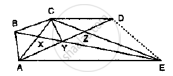Ex. 14.30 | Q 22 | Page 47

In below fig., PSDA is a parallelogram in which PQ = QR = RS and AP || BQ || CR. Prove
that ar (Δ PQE) = ar (ΔCFD).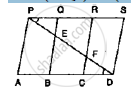Ex. 14.30 | Q 23 | Page 47

In the below fig. ABCD is a trapezium in which AB || DC and DC = 40 cm and AB = 60
cm. If X and Y are respectively, the mid-points of AD and BC, prove that:
(i) XY = 50 cm
(ii) DCYX is a trapezium
(iii) ar (trap. DCYX) =9/11ar (trap. (XYBA))

Ex. 14.30 | Q 24 | Page 47

D is the mid-point of side BC of ΔABC and E is the mid-point of BD. if O is the mid-point
of AE, prove that ar (ΔBOE) = 1/8 ar (Δ ABC).

Ex. 14.30 | Q 25 | Page 47

In the below fig. X and Y are the mid-points of AC and AB respectively, QP || BC and
CYQ and BXP are straight lines. Prove that ar (Δ ABP) = ar (ΔACQ).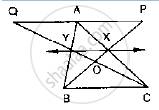Ex. 14.30 | Q 26 | Page 47

In the below fig. ABCD and AEFD are two parallelograms. Prove that
(1) PE = FQ
(2) ar (Δ APE) : ar (ΔPFA) = ar Δ(QFD) : ar (Δ PFD)
(3) ar (ΔPEA) = ar (ΔQFD)

Ex. 14.30 | Q 27 | Page 48

In the below figure, ABCD is parallelogram. O is any point on AC. PQ || AB and LM ||
AD. Prove that ar (||gm DLOP) = ar (||gm BMOQ)

Ex. 14.30 | Q 28 | Page 48

In a ΔABC, if L and M are points on AB and AC respectively such that LM || BC. Prove
that:

(1) ar (ΔLCM ) = ar (ΔLBM )
(2) ar (ΔLBC) = ar (ΔMBC)
(3) ar (ΔABM) ar (ΔACL)
(4) ar (ΔLOB) ar (ΔMOC)

Ex. 14.30 | Q 29 | Page 48

In Fig. below, ABC and BDE are two equilateral triangles such that D is the mid-point of
BC. AE intersects BC in F. Prove that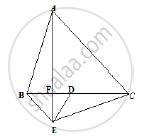(1)  ar (Δ BDE) = 1/2 ar (ΔABC)

(2) Area ( ΔBDE) = 1/2  ar (ΔBAE)

(3)  ar (BEF) = ar (ΔAFD)

(4) area (Δ ABC) = 2 area (ΔBEC)

(5) ar (ΔFED) = 1/8 ar (ΔAFC)

(6) ar (Δ BFE) = 2 ar (ΔEFD)

Ex. 14.30 | Q 30 | Page 48

In the following figure, ABC is a right triangle right angled at A. BCED, ACFG and ABMN are squares on the sides BC, CA and AB respectively. Line segment AX ⊥ DE meets BC at Y. Show that:-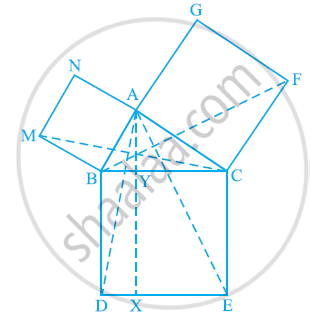(i) ΔMBC ≅ ΔABD

(ii) ar (BYXD) = 2 ar(MBC)

(iii) ar (BYXD) = ar(ABMN)

(iv) ΔFCB ≅ ΔACE

(v) ar(CYXE) = 2 ar(FCB)

(vi) ar (CYXE) = ar(ACFG)

(vii) ar (BCED) = ar(ABMN) + ar(ACFG)

Note : Result (vii) is the famous Theorem of Pythagoras. You shall learn a simpler proof of this theorem in Class X.

Chapter 14: Areas of Parallelograms and Triangles solutions [Pages 59 - 60]

Q 1 | Page 59

If ABC and BDE are two equilateral triangles such that D is the mid-point of BC, then find ar (ΔABC) : ar (ΔBDE).

Q 2 | Page 59

In the given figure, ABCD is a rectangle in which CD = 6 cm, AD = 8 cm. Find the area of parallelogram CDEF.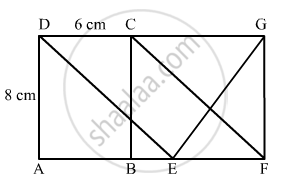Q 3 | Page 59

In the given figure, find the area of ΔGEF.

Q 4 | Page 59

In the given figure, ABCD is a rectangle with sides AB = 10 cm and AD = 5 cm. Find the area of ΔEFG.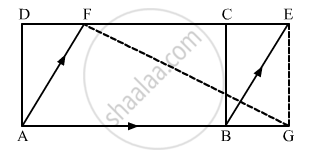Q 5 | Page 60

PQRS is a rectangle inscribed in a quadrant of a circle of radius 13 cm. A is any point on PQ. If PS = 5 cm, then find ar (ΔRAS)

Q 6 | Page 60

In square ABCDP and Q are mid-point of AB and CD respectively. If AB = 8cm and PQand BD intersect at O, then find area of ΔOPB.

Q 7 | Page 60

ABC is a triangle in which D is the mid-point of BCand F are mid-points of DC and AErespectively. IF area of ΔABC is 16 cm2, find the area of ΔDEF.

Q 8 | Page 60

PQRS is a trapezium having PS and QR as parallel sides. A is any point on PQ and is a point on SR such that AB || QR. If area of ΔPBQ is 17cm2, find the area of ΔASR.

Q 9 | Page 60

ABCD is a parallelogram. P is the mid-point of ABBD and CP intersect at Q such that CQQP = 3.1. If ar (ΔPBQ) = 10cm2, find the area of parallelogram ABCD.

Q 10 | Page 60

P is any point on base BC of ΔABC and D is the mid-point of BCDE is drawn parallel toPA to meet AC at E. If ar (ΔABC) = 12 cm2, then find area of ΔEPC

Chapter 14: Areas of Parallelograms and Triangles solutions [Pages 60 - 62]

Q 1 | Page 60

Two parallelograms are on the same base and between the same parallels. The ratio of their areas is

•  1 : 2

•  2 : 1

•  1 : 1

•  3 : 1

Q 2 | Page 60

A triangle and a parallelogram are on the same base and between the same parallels. The ratio of the areas of triangle and parallelogram is

• 1 : 1

• 1 : 2

• 2 : 1

•  1 : 3

Q 3 | Page 60

Let ABC be a triangle of area 24 sq. units and PQR be the triangle formed by the mid-points of the sides of Δ ABC. Then the area of ΔPQR is

• 12 sq. units

•  6 sq. units

• 4 sq. units

• 3 sq. units

Q 4 | Page 60

The median of a triangle divides it into two

• congruent triangle

•  isosceles triangles

•  right triangles

•  triangles of equal areas

Q 5 | Page 60

In a ΔABCDEF are the mid-points of sides BCCA and AB respectively. If ar (ΔABC) = 16cm2, then ar (trapezium FBCE) =

•  4 cm2

•  8 cm2

• 12 cm2

• 10 cm2

Q 6 | Page 60

ABCD is a parallelogram. P is any point on CD. If ar (ΔDPA) = 15 cm2 and ar (ΔAPC) = 20 cm2, then ar (ΔAPB) =

• 15 cm2

• 20 cm2

• 35 cm2

• 30 cm2

Q 7 | Page 61

The area of the figure formed by joining the mid-points of the adjacent sides of a rhombus with diagonals 16 cm and 12 cm is

•  28 cm2

•  48 cm2

• 96 cm2

• 24 cm2

Q 8 | Page 61

A, B, C, D are mid-points of sides of parallelogram PQRS. If ar (PQRS) = 36 cm2, then ar (ABCD) =

•  24 cm2

•  18cm2

•  30 cm2

•  36 cm2

Q 9 | Page 61

The figure obtained by joining the mid-points of the adjacent sides of a rectangle of sides 8 cm and 6 cm is

•  a rhombus of area 24 cm2

• a rectangle of area 24 cm2

• a square of area 26 cm2

•  a trapezium of area 14 cm2

Q 10 | Page 61

If AD is median of ΔABC and is a point on AC such that
ar (ΔADP) : ar (ΔABD) = 2 : 3, then ar (Δ PDC) : ar (Δ ABC)

• 1 : 5

• 1 : 5

• 1 : 6

• 3 : 5

Q 11 | Page 61

Medians of ΔABC intersect at G. If ar (ΔABC) = 27 cm2, then ar (ΔBGC) =

• 6 cm2

•  9 cm2

• 12 cm2

•  18 cm2

Q 12 | Page 61

In a ΔABC if D and E are mid-points of BC and AD respectively such that ar (ΔAEC) = 4cm2, then ar (ΔBEC) =

• 4 cm2

• 6 cm2

•  8 cm2

• 12 cm2

Q 13 | Page 61

In the given figure, ABCD is a parallelogram. If AB = 12 cm, AE = 7.5 cm, CF = 15 cm, then AD =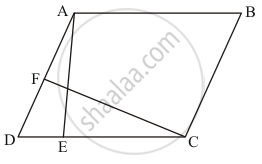Q 14 | Page 61

In the given figure, PQRS is a parallelogram. If X and Y are mid-points of PQ and SRrespectively and diagonal Q is joined. The ratio ar (||gm XQRY) : ar (ΔQSR) =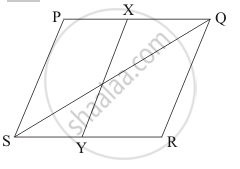•  1 : 4

•  2 : 1

•  1 : 2

• 1 : 1

Q 15 | Page 61

Diagonal AC and BD of trapezium ABCD, in which AB || DC, intersect each other at O. The triangle which is equal in area of ΔAOD is

•  ΔAOB

•  ΔBOC

• ΔDOC

Q 16 | Page 61

ABCD is a trapezium in which AB || DC. If ar (ΔABD) = 24 cm2 and AB = 8 cm, then height of ΔABC is

•  3 cm

• 4 cm

•  6 cm

•  8 cm

Q 17 | Page 62

ABCD is a trapezium with parallel sides AB =a and DC = b. If E and F are mid-points of non-parallel sides AD and BC respectively, then the ratio of areas of quadrilaterals ABFEand EFCD is

• a : b

• (a + 3b): (3a + b)

• (3a + b) : (a + 3b)

•  (2a + b) : (3a + b)

Q 18 | Page 62

ABCD is a rectangle with O as any point in its interior. If ar (ΔAOD) = 3 cm2 and ar (ΔABOC) = 6 cm2, then area of rectangle ABCD is

• 9 cm2

• 12 cm2

• 15 cm2

• 18 cm2

Q 19 | Page 62

The mid-points of the sides of a triangle ABC along with any of the vertices as the fourth point make a parallelogram of area equal to

•  ar (ΔABC)

• $\frac{1}{2}$ ar (ΔABC)

• $\frac{1}{3}$ ar (ΔABC)

• $\frac{1}{4}$  ar (ΔABC)

Q 20 | Page 62

In the given figure, ABCD and FECG are parallelograms equal in area. If ar (ΔAQE) = 12 cm2, then ar (||gm FGBQ) =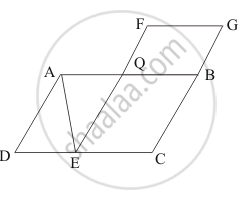•  12 cm2

•  20 cm2

• 24 cm2

•  36 cm2

Chapter 14: Areas of Parallelograms and Triangles

Ex. 14.10Ex. 14.20Ex. 14.30Others

RD Sharma Mathematics Class 9 by R D Sharma (2018-19 Session)RD Sharma solutions for Class 9 Mathematics chapter 14 - Areas of Parallelograms and Triangles

RD Sharma solutions for Class 9 Maths chapter 14 (Areas of Parallelograms and Triangles) include all questions with solution and detail explanation. This will clear students doubts about any question and improve application skills while preparing for board exams. The detailed, step-by-step solutions will help you understand the concepts better and clear your confusions, if any. Shaalaa.com has the CBSE Mathematics for Class 9 by R D Sharma (2018-19 Session) solutions in a manner that help students grasp basic concepts better and faster.

Further, we at Shaalaa.com are providing such solutions so that students can prepare for written exams. RD Sharma textbook solutions can be a core help for self-study and acts as a perfect self-help guidance for students.

Concepts covered in Class 9 Mathematics chapter 14 Areas of Parallelograms and Triangles are Triangles on the Same Base and Between the Same Parallels, Introduction to Areas of Parallelograms and Triangles, Figures on the Same Base and Between the Same Parallels, Parallelograms on the Same Base and Between the Same Parallels.

Using RD Sharma Class 9 solutions Areas of Parallelograms and Triangles exercise by students are an easy way to prepare for the exams, as they involve solutions arranged chapter-wise also page wise. The questions involved in RD Sharma Solutions are important questions that can be asked in the final exam. Maximum students of CBSE Class 9 prefer RD Sharma Textbook Solutions to score more in exam.

Get the free view of chapter 14 Areas of Parallelograms and Triangles Class 9 extra questions for Maths and can use Shaalaa.com to keep it handy for your exam preparation

S